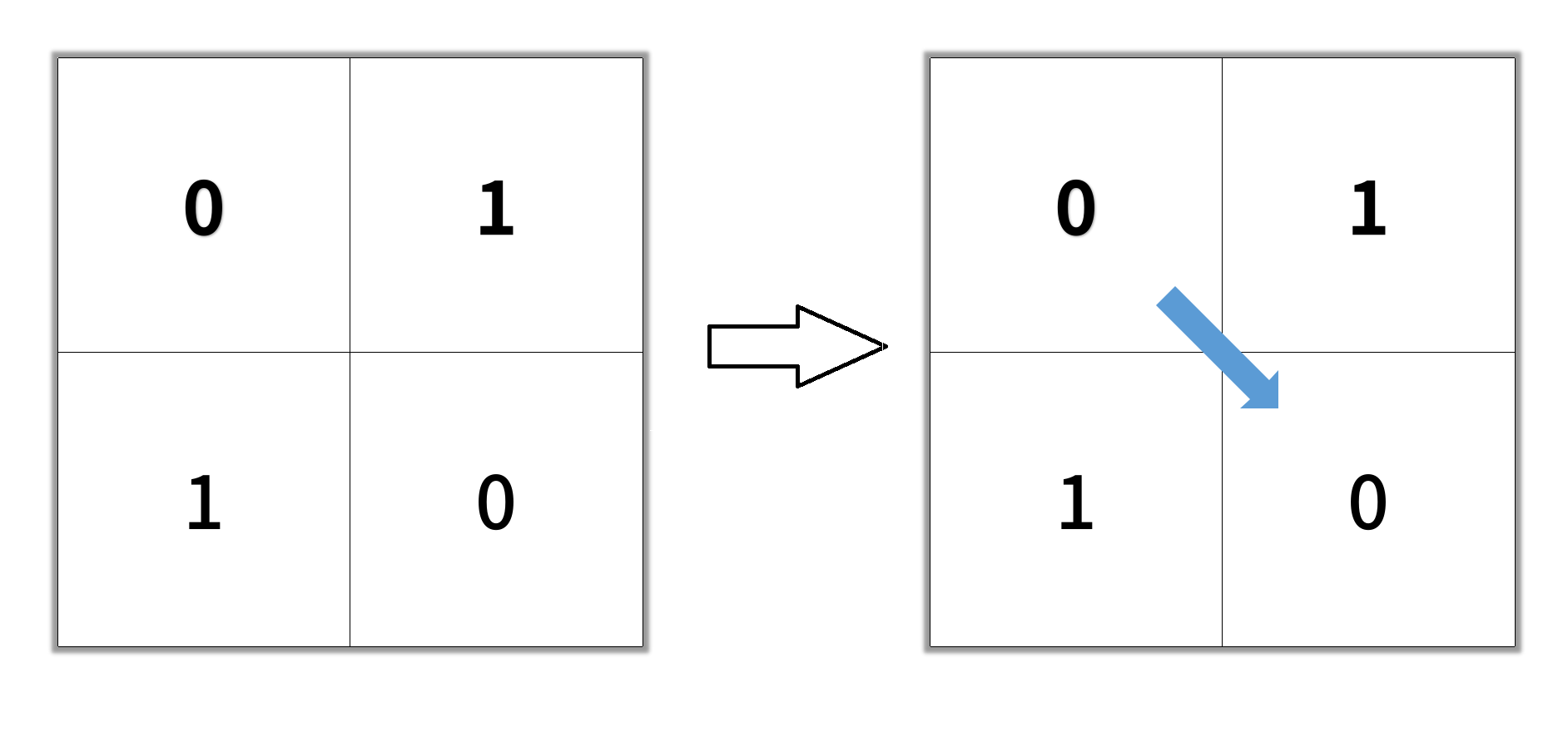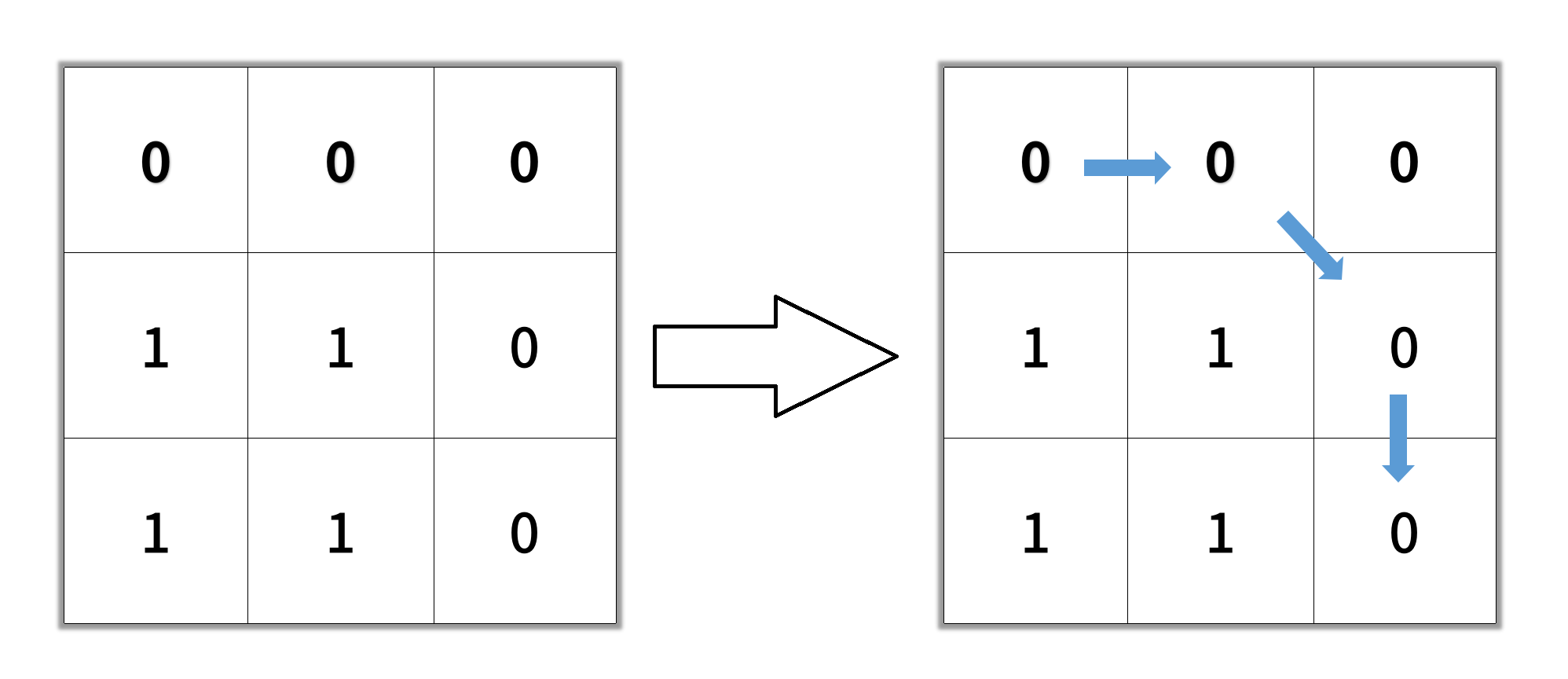# LeetCode Shortest Path in Binary Matrix Solution

Given an `n x n` binary matrix `grid`, return the length of the shortest clear path in the matrix. If there is no clear path, return `-1`.

A clear path in a binary matrix is a path from the top-left cell (i.e., `(0, 0)`) to the bottom-right cell (i.e., `(n - 1, n - 1)`) such that:

• All the visited cells of the path are `0`.
• All the adjacent cells of the path are 8-directionally connected (i.e., they are different and they share an edge or a corner).

The length of a clear path is the number of visited cells of this path.

Example 1:Input: `grid = [[0,1],[1,0]]`
Output: `2`

Example 2:Input: `grid = [[0,0,0],[1,1,0],[1,1,0]]`
Output: `4`

Example 3:
Input: `grid = [[1,0,0],[1,1,0],[1,1,0]]`
Output: `-1`

Constraints:

• `n == grid.length`
• `n == grid[i].length`
• `1 <= n <= 100`
• `grid[i][j] is 0 or 1`

## Solution

To find the shortest clear path from (0,0) to (n-1, n-1), we will perform breadth first search from (0,0). First, we need to make sure that `grid` is `0`, otherwise a clear path does not exist. Each cell has (at most) 8 neighbours, and we can use the `get_neighbours` function to find the neighbours. Then, we will only continue on cells labeled `0`, the number of levels of the BFS traversal is the length of the shortest clear path.

#### Implementation

``````1def shortestPathBinaryMatrix(self, grid: List[List[int]]) -> int:
2    if grid == 1: return -1
3
4    n = len(grid)
5    dirs = [(-1, -1), (-1, 0), (-1, 1), (0, -1), (0, 1), (1, -1), (1, 0), (1, 1)]
6    def get_neighbours(x, y):
7        neighbours = []
8        for dx, dy in dirs:
9            if 0 <= x+dx < n and 0 <= y+dy < n:
10                neighbours.append((x+dx, y+dy))
11        return neighbours
12
13    length = 1
14    q = deque()
15    q.append((0,0))
16    unvisited = [[True for _ in range(n)] for _ in range(n)]
17    unvisited = False
18    while q:
19        count = len(q)
20        for _ in range(count):
21            x, y = q.popleft()
22            if x == n -1 and y == n-1:
23                return length
24            for nx, ny in get_neighbours(x, y):
25                if grid[nx][ny] == 0 and unvisited[nx][ny]:
26                    q.append((nx, ny))
27                    unvisited[nx][ny] = False
28        length += 1
29    return -1``````